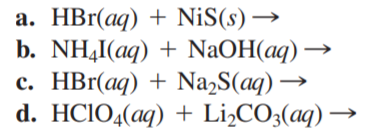×
Get Full Access to Chemistry: A Molecular Approach - 3 Edition - Chapter 4 - Problem 89e
Get Full Access to Chemistry: A Molecular Approach - 3 Edition - Chapter 4 - Problem 89e

×

# Solved: Complete and balance each gas-evolution equation.ISBN: 9780321809247 1

## Solution for problem 89E Chapter 4

Chemistry: A Molecular Approach | 3rd Edition

• Textbook Solutions
• 2901 Step-by-step solutions solved by professors and subject experts
• Get 24/7 help from StudySoup virtual teaching assistantsChemistry: A Molecular Approach | 3rd Edition

4 5 1 369 Reviews
29
4
Problem 89E

Complete and balance each gas-evolution equation.Step-by-Step Solution:
Step 1 of 3

Solution: Here, we are going to calculate the percentage by mass of magnesium hydroxide in the given sample. Step1: Given, the volume of NaOH solution used = 19.85 mL = 19.85 / 1000 L = 0.01985 L The molarity of NaOH solution used = 0.1020 M Therefore, number of moles of NaOH solution = Volume of solution in litres X Molarity = 0.01985 L X 0.1020 M = 0.002 mol Step2: Again, the volume of HCl solution used = 100.0 mL = 100 / 1000 L = 0.100 L The molarity of HCl solution used = 0.2050 M Therefore, number of moles of HCl solution = Volume of solution in litres X Molarity = 0.100 L X 0.2050 M = 0.0205 mol Since, 1 mole of NaOH will react with 1 mole of HCl for complete neutralisation, therefore, 0.002 mol of NaOH will react will 0.002 mol of HCl for neutralization. Thus, number of moles of HCl reacted with magnesium hydroxide = total number of moles of HCl - number of moles of HCl that reacted with NaOH = (0.0205 - 0.002) mol = 0.0185 mol Step3: The reaction between HCl and Mg(OH) takes place in the following way: 2 2HCl(aq) + Mg(OH) (aq) 2-------> MgCl (aq) + 2 O 2 From the above equation it is clear that, 2 moles of HCl reacts with 1 mole of Mg(OH) for 2 complete neutralisation. Therefore, 0.0185 mol of HCl will react with (0.0185 / 2 = 0.00925) mol of Mg(OH) for 2 complete neutralisation. Step4: Now, number of moles of Mg(OH) = mass of Mg(OH) / molar mass 2 2 Therefore, mass of Mg(OH) used =2umber of moles X molar mass = 0.00925 X 58.32 g [molar mass of Mg(OH) = 58.32 2 g/mol] = 0.539 g Thus mass percentage of Mg(OH) = (mass of Mg(2) used / total mass of Mg2H) ) X 100 2 = (0.539 / 0.5895) X 100 % = 0.914 X 100 % = 91.4 % -------------------

Step 2 of 3

Step 3 of 3

##### ISBN: 9780321809247

Since the solution to 89E from 4 chapter was answered, more than 436 students have viewed the full step-by-step answer. This textbook survival guide was created for the textbook: Chemistry: A Molecular Approach, edition: 3. This full solution covers the following key subjects: hydroxide, solution, sample, magnesium, mass. This expansive textbook survival guide covers 82 chapters, and 9454 solutions. The full step-by-step solution to problem: 89E from chapter: 4 was answered by , our top Chemistry solution expert on 02/22/17, 04:35PM. Chemistry: A Molecular Approach was written by and is associated to the ISBN: 9780321809247. The answer to “?Complete and balance each gas-evolution equation.” is broken down into a number of easy to follow steps, and 6 words.

## Discover and learn what students are asking

Chemistry: The Central Science : Atoms, Molecules, and Ions
?Are these two compounds isomers? Explain. [Section 2.9]

Unlock Textbook Solution